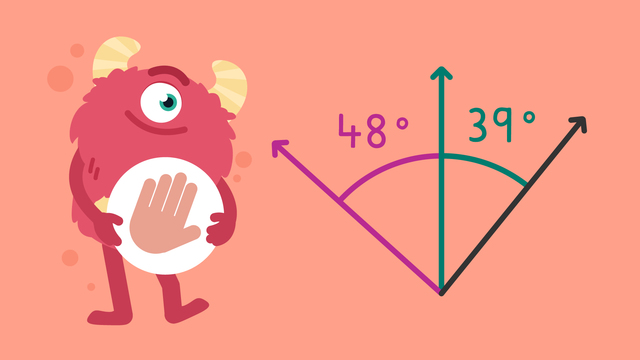# Additive Angles — Let's Practice!Rating

Ø 5.0 / 3 ratings
The authorsTeam Digital
CCSS.MATH.CONTENT.4.MD.C.7

## Basics on the topicAdditive Angles — Let's Practice!

Today we are practicing additive angles with Razzi! This video contains examples to help you further practice and grow confident in this area.

## Additive Angles — Let's Practice! exercise

Would you like to apply the knowledge you’ve learned? You can review and practice it with the tasks for the video Additive Angles — Let's Practice! .
• ### Find the combined angles.

Hints

In this example, to find the combined angles we add 20 + 45.

When adding the two angles, try adding the ones first and then the tens.

Solution
1. 31° + 52° = 83°
2. 39° + 47° = 86°
3. 47° + 71° = 118°
4. 52° + 32° = 84°
• ### What is the total of the combined angles?

Hints

To find the total, all three angles must be added together.

Try partitioning each number and adding the tens and ones separately.

First add two angles together, then add the third angle on to this total.

Solution

26° + 52° + 32° = 110°

• ### What is the total of the two angles?

Hints

First find the value of the two angles that must be added together.

Angle (a) is 36° and angle (b) is 42°, now we need to add these together to find the combined amount.

Solution

36° + 42° = 78°.

• ### What do the angles total?

Hints

There are three angles that we need to add together.

Try adding the tens and ones separately, for example, first add 70 + 60 + 20.

Solution

75° + 60° + 24° = 159°.

We could solve this by first adding the tens: 70 + 60 + 20 = 150 then adding the ones, 5 + 0 + 4 = 9 before combining these two answers together. 150 + 9 = 159°.

• ### What is the sum of the combined angles?

Hints

To find the combined angles, the two separate angles need to be added together.

What is 38 + 22?

Try adding the ones first and then the tens.

Solution

38° + 22° = 60°

• ### Find the missing angle.

Hints

We know that (a) + (b) + (c) = 152. What is the total of (a) + (c)?

(a) + (c) or 35° + 78° = 113°. What do we need to do next to find angle (b)?

152 - 113 will give the answer to the missing angle (b).

Solution
• (a) + (b) + (c) = 152°
• (a) + (c) = 113°
• 152 - 113 = 39°
• We can check this by adding all three angles together: 35 + 39 + 78 = 152.